Function Repository Resource:

# PulsarPDotDiagram

Plot the positions of pulsars in a P-Ṗ diagram

Contributed by: Jeff Bryant
 ResourceFunction["PulsarPDotDiagram"][entities] plots the positions of the specified entities on a P‐Ṗ diagram.

## Details and Options

When the rotation period (P) of a pulsar is plotted versus its rotation period derivative (), its position on the resulting diagram can provide useful classification information.
Valid domains for entities or entity classes must be from the "Pulsar" domain.

## Examples

### Basic Examples (3)

Plot single pulsars on the P diagram:

 In:=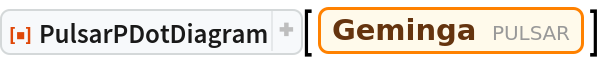Out=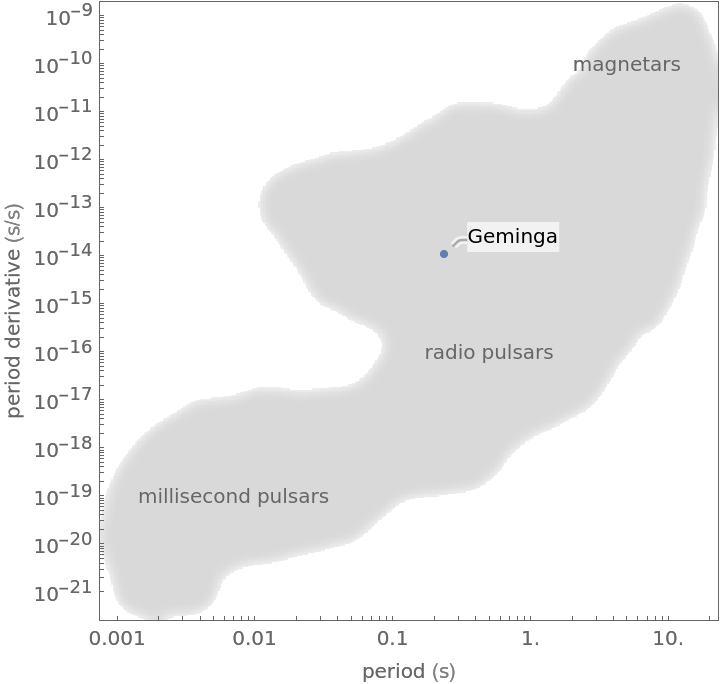Plot multiple pulsars on the P diagram:

 In:=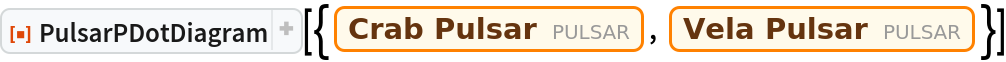Out=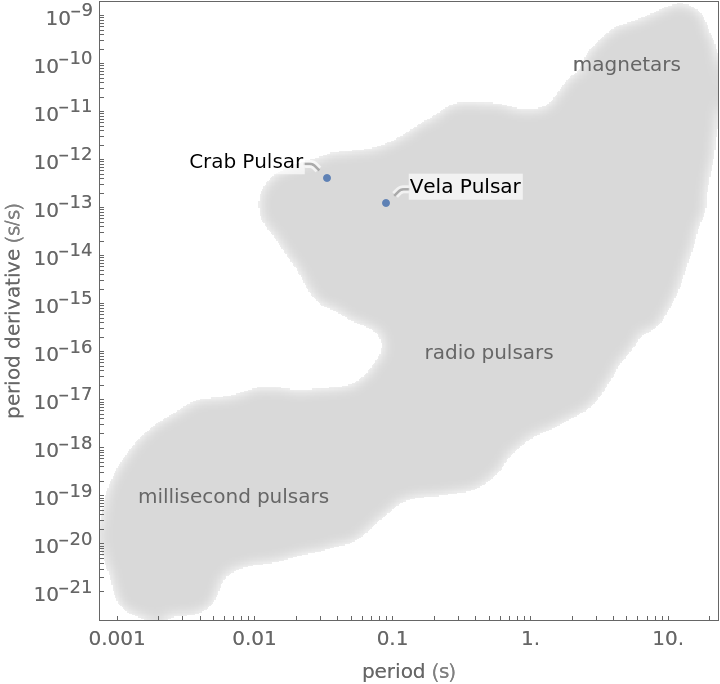Plot entity classes of pulsars on the P diagram:

 In:=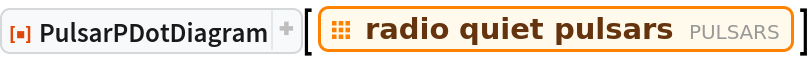Out=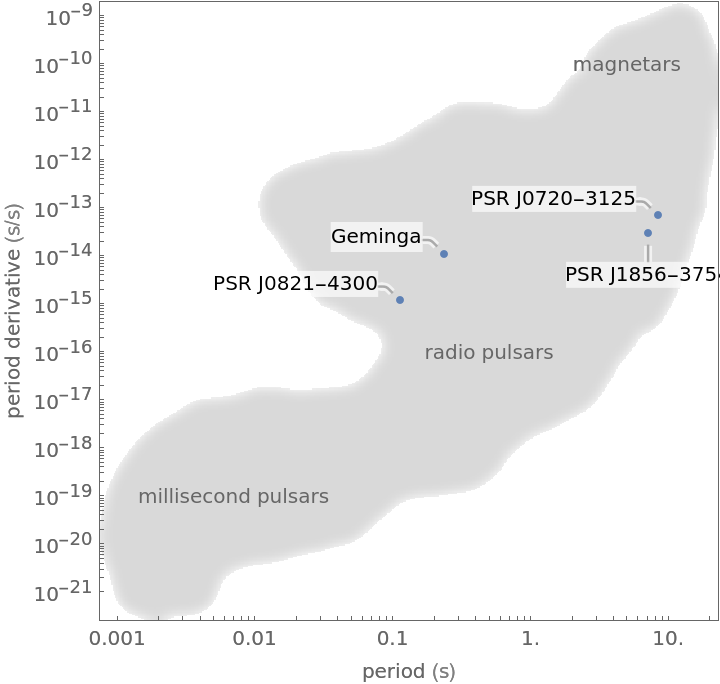### Options (2)

Change the color of the pulsar(s) with the option PlotStyle:

 In:=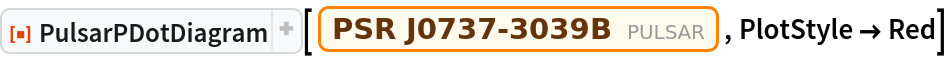Out=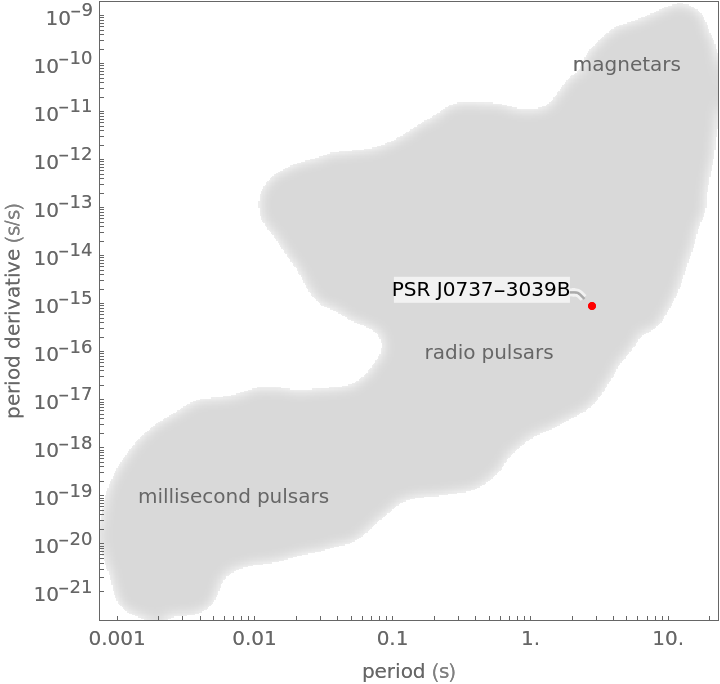Change the formatting of the background with the option PlotTheme:

 In:=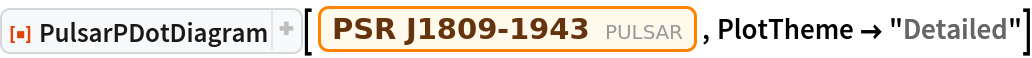Out=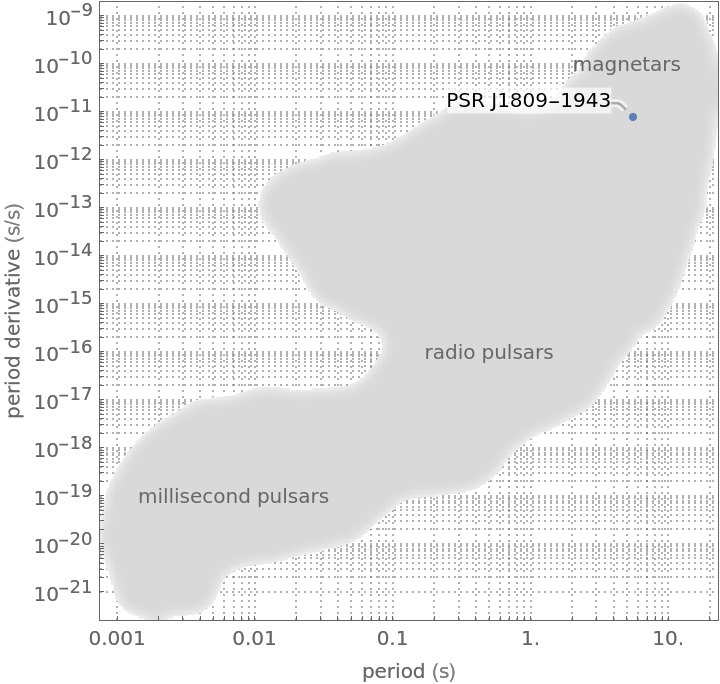### Applications (2)

Binary pulsars are correlated with millisecond pulsars which are "spun up" due to mass transfer, decreasing the rotation period:

 In:=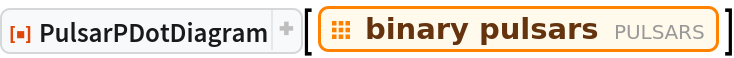Out=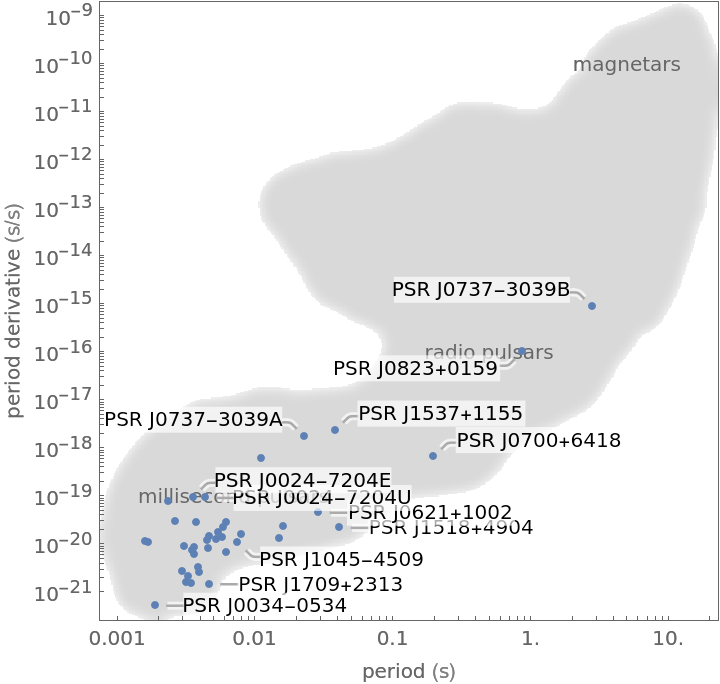Magnetars undergo more magnetic braking and thus a higher rotation period derivative as they slow down with age along with slower rotation compared to other pulsars:

 In:=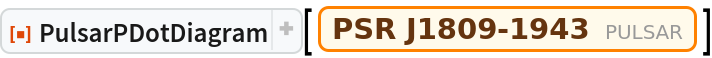Out=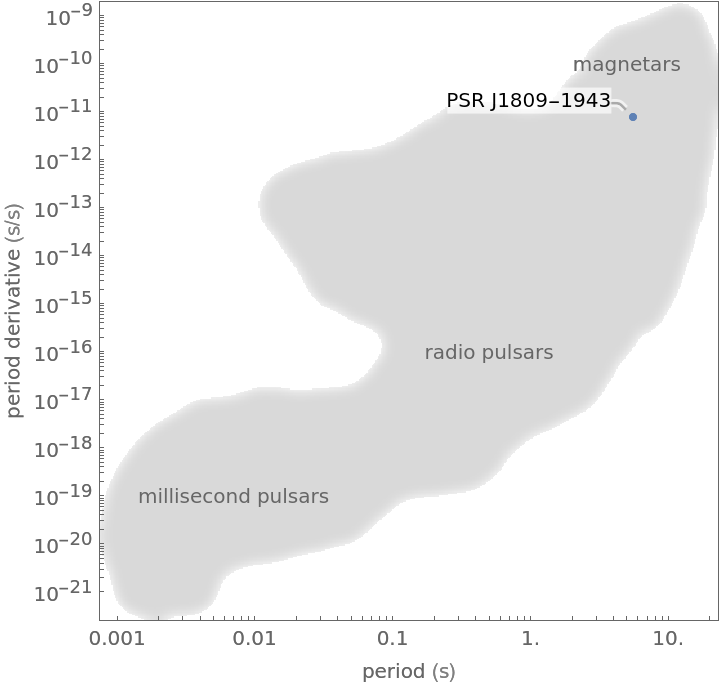## Version History

• 1.0.0 – 10 June 2022